Next: The Sky Grid Up: Sky Exposure Previous: Sky Exposure   Contents

#### Orbit Grid

In order to study the visibility of each single sky direction as a function of time, we analysed all the BeppoSAX ephemerides in the time interval: July 3, 1996 - October 3, 2001. Let (X,Y,Z) the BeppoSAX position vector referred to a geocentric frame of reference; let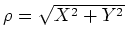be the XY distance from the Earth center,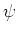the angle between the (X,Y,0) and the (1,0,0) directions; the cylindrical variables (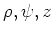) have been found to vary within the following ranges: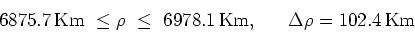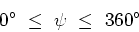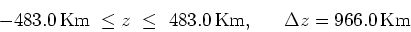The rectangular section torus containing all the BeppoSAX orbits has been split into a grid of cells of coordinates (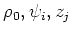),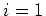,...,360,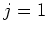,...,8. Taking a fixed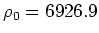Km, i.e. the mean value of the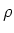range, an angular step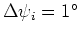, and astep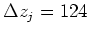Km, the resulting grid consists of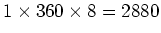cells, that can be approximated to parallelepipedal (nearly cubic) (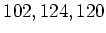) Km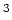blocks ().

Whenever the spacecraft is inside a given cell, its position is approximated to the cell center, whose coordinates are expressed as follows: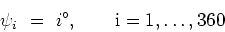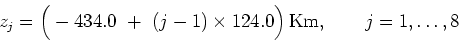The step between two adjacent cells (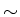120 Km) subtends an angle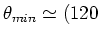Km)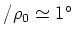with respect to the Earth center; hence, the angular resolution of the sky portion which is calculated to be Earth-blocked is, when using such an orbit grid.Next: The Sky Grid Up: Sky Exposure Previous: Sky Exposure   Contents
Cristiano Guidorzi 2003-07-31